Next: Criticism of the conventional interpretation Up: Notions of relativistic dynamics Previous: The Maxwell equations   Contents

In the classical physics all concepts have a clearly definite sense, and they should not be replaced with ersatzes. Let the relativists be inventing other names to their new concepts (or, more correctly to combinations of symbols). The relativistic definition of coordinates of the center of inertia :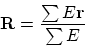has no physical sense, since in SRT the center of inertia of the same system of moving particles occurs to be different in various frames of reference. Therefore, it does not fulfill its functional designation of the center of equilibrium. Let we have a massive planar box, in which the massive balls are moving. Let in the classical case the center of inertia of the whole system (in the course of motion and collisions of balls) be always coinciding with the center of a box. Then in the classical case we can balance it (for example, in the Earth's field of gravity or in some other field) on a support of small cross section (Fig. 4.3), and the equilibrium will be kept.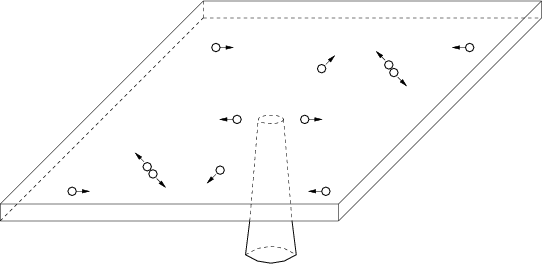In SRT, on the opposite, if we only shall look at this system from a rapidly moving relativistic missile, then the center of inertia can appear to be not above a support, and the equilibrium will be violated. A remarkable objectivity of SRT: in order that the equilibrium of plasma in a controlled thermonuclear fusion not be disturbed, we ask the relativistic missiles not to fly and not to "spy" upon the experiment.

The relativistic bond of the mass and energy actually reflects no principal thing. Indeed, the classical expression for kinetic energy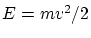and the relativistic expression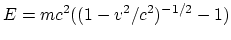do not differ in any (qualitatively) significant thing. Both these quantities are calculated quantities. The attempt to measure these quantities (that is, the calibration of an instrument) depends on interpretation of the theory, since these quantities can not be determined from the comparison with a measurement standard. Since the relativistic expression of energy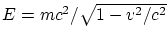includes, except the mass, the other quantities, then for any possible interrelations the mass and energy will remain different (nonequivalent, independent) quantities. Even for the so-called "energy of rest"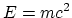the question can not be about mutual transformations of mass and energy. The fact is, that at annihilation (the only "candidate" for a similar process) the photons are generated, for which the "mass of motion" is postulated in SRT according to the same formula. Therefore, in this case the question is simply about mutual transformations of particles too. To say nothing of the fact, that the "energy of rest" is only the hypothesis of SRT, because this theory leads again to the same indeterminate constant, as in the classical physics.

We call attention to a non-invariance of the formulain framework of SRT: mass is invariant, light speed is also invariant. However, energy represents by itself a four-vector. If the kinetic energy of molecules, which move with different velocities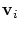, is included in the full energy of a body, then these velocities will be added up in different manner with the velocity of the body as a whole in other moving system. As the result, the relationship will be violated and in new system this formula will be simple some relativistic definition for some "letter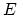".

SRT tries to fight, from principal grounds, "against the windmills": for example, against the notion of absolute rigid body. In the classical physics, however, nobody assigns a literal sense in the abstraction of absolutely rigid body. It is obvious for everybody, that there are no absolutely rigid bodies even at absolutely non-relativistic velocities (we shall mention the role of accelerations, or, more correctly, of forces, in this issue by remembering usual collisions of cars on roads). Simply, in describing some motions the influence of strains is negligible or unessential for the phenomenon under study, and then, only for the sake of simplifying mathematical derivations, the absolutely rigid body abstraction is applied. SRT principally tries to consider elementary particles to be points  and immediately encounters another principal problem - the singularity of some quantities.

Now we shall directly pass to remarks on relativistic dynamics (the theory of collisions and laws of motion of charged particles).Next: Criticism of the conventional interpretation Up: Notions of relativistic dynamics Previous: The Maxwell equations   Contents
Sergey N. Arteha